# Selina Solutions Concise Maths Class 10 Chapter 7 Ratio and Proportion Exercise 7(D)

Different models of problems based on the concepts of the entire chapter are listed in this exercise. Additionally, duplicate, triplicate, sub-duplicate and sub-triplicate ratio are some of the key insights in the exercise. The Selina Solutions for Class 10 Maths can be used by students for any quick references as well as a guide for students in order to help their exam preparation. The solutions to this exercise can be accessed in the Concise Selina Solutions for Class 10 Maths Chapter 7 Ratio and Proportion Exercise 7(D) PDF, which is provided in the links below.

## Selina Solutions Concise Maths Class 10 Chapter 7 Ratio and Proportion Exercise 7(D) Download PDF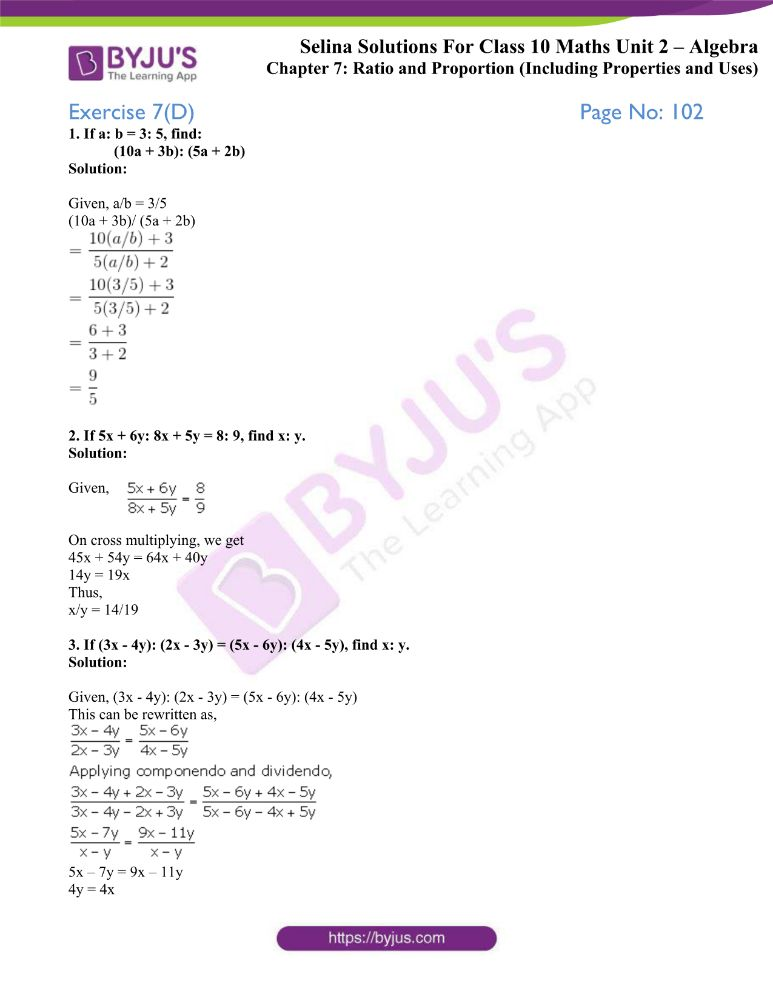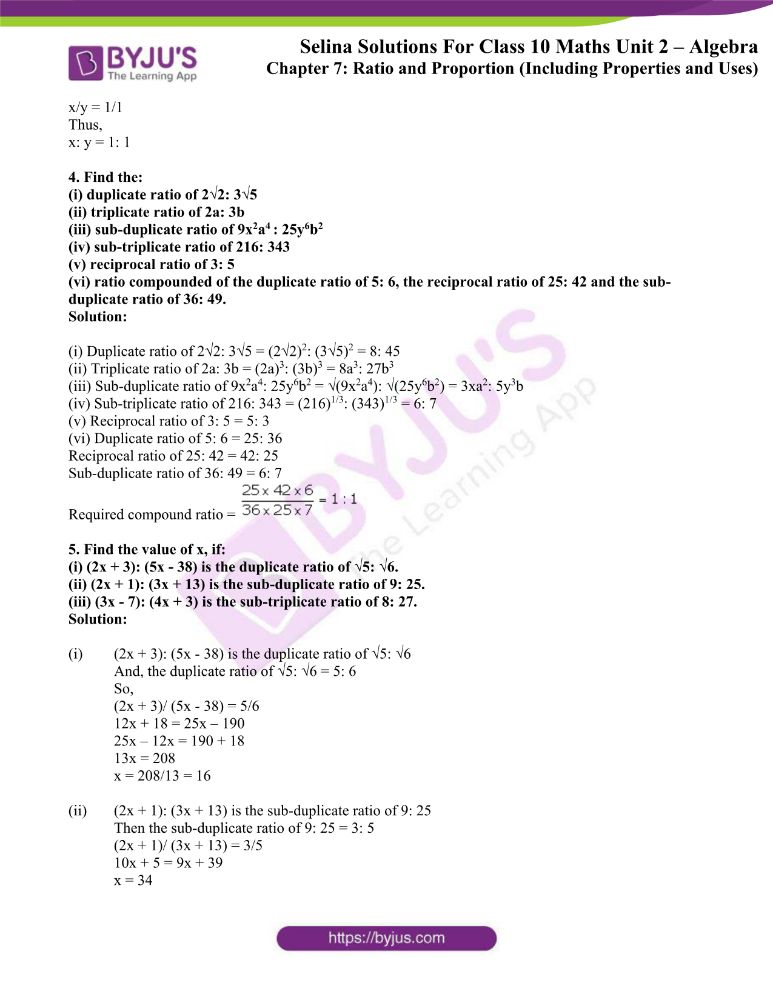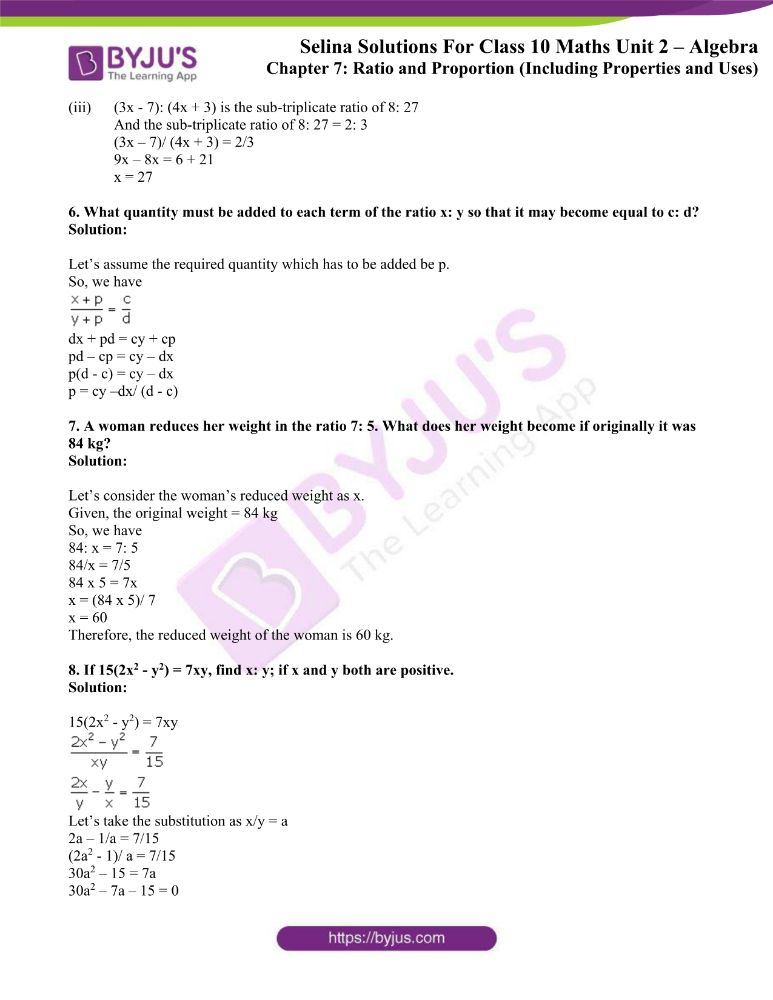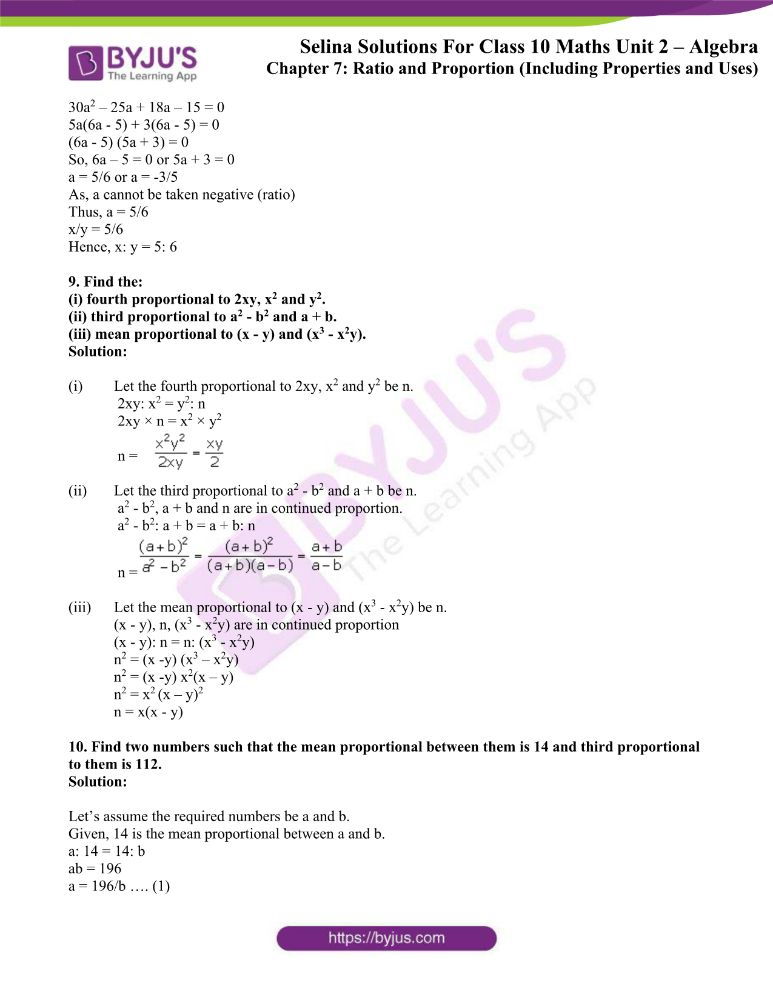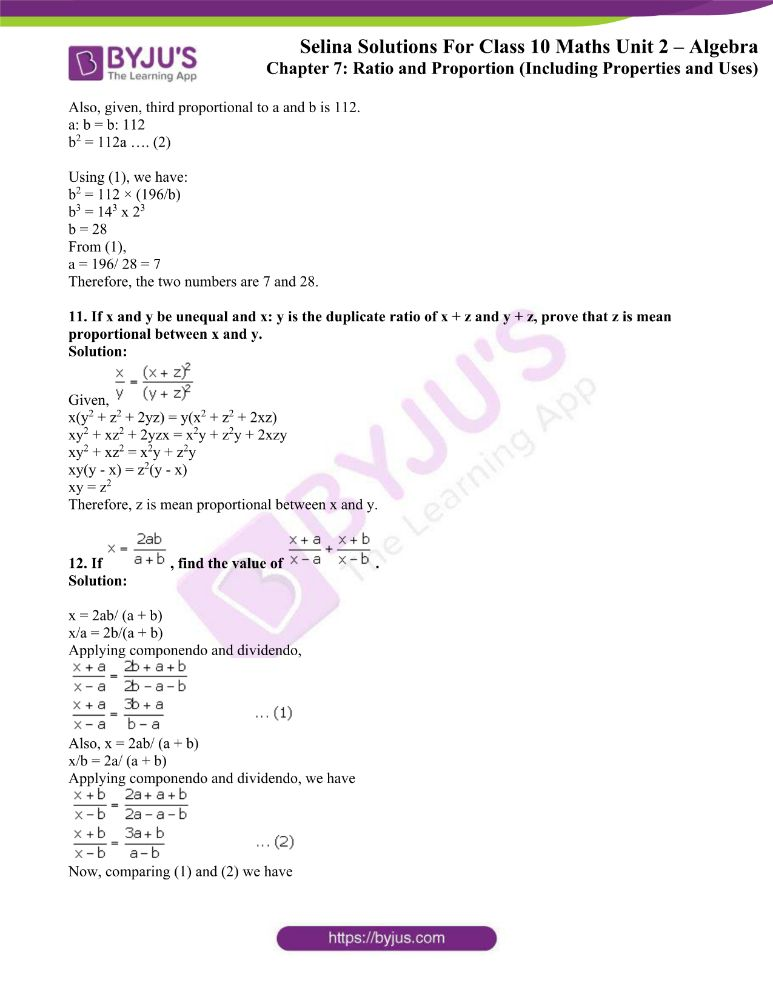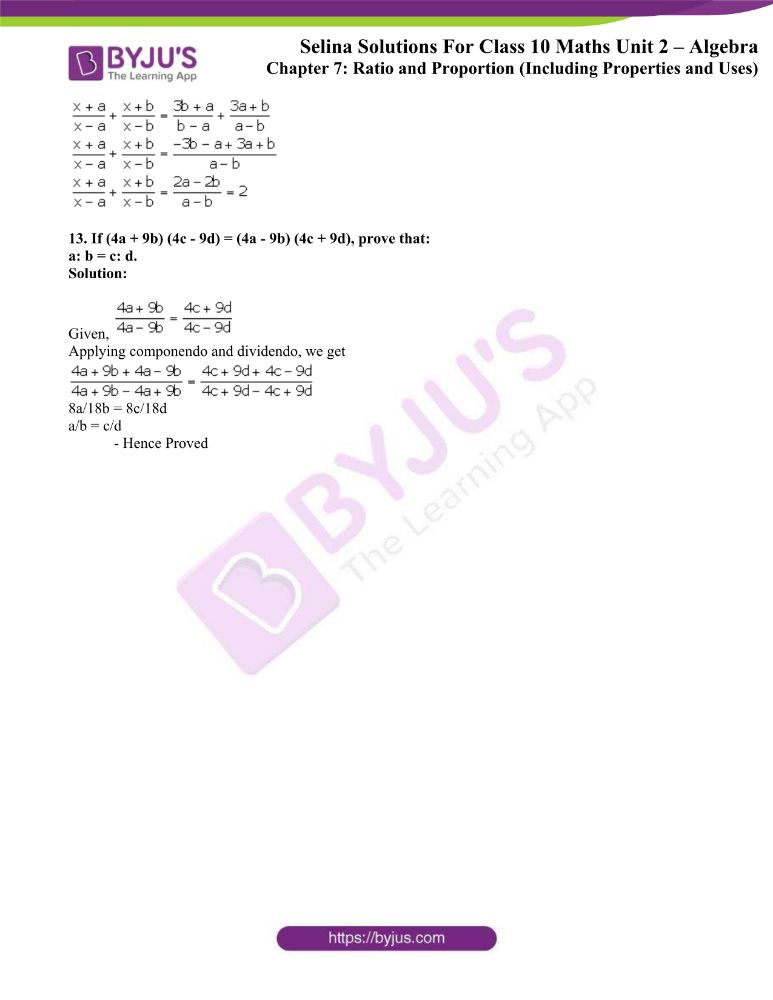### Access other exercises of Selina Solutions Concise Maths Class 10 Chapter 7 Ratio and Proportion

Exercise 7(A) Solutions

Exercise 7(B) Solutions

Exercise 7(C) Solutions

### Access Selina Solutions Concise Maths Class 10 Chapter 7 Ratio and Proportion Exercise 7(D)

1. If a: b = 3: 5, find:

(10a + 3b): (5a + 2b)

Solution:

Given, a/b = 3/5

(10a + 3b)/ (5a + 2b)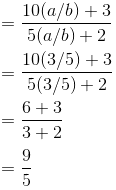2. If 5x + 6y: 8x + 5y = 8: 9, find x: y.

Solution: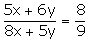Given,

On cross multiplying, we get

45x + 54y = 64x + 40y

14y = 19x

Thus,

x/y = 14/19

3. If (3x – 4y): (2x – 3y) = (5x – 6y): (4x – 5y), find x: y.

Solution:

Given, (3x – 4y): (2x – 3y) = (5x – 6y): (4x – 5y)

This can be rewritten as,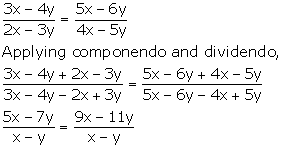5x – 7y = 9x – 11y

4y = 4x

x/y = 1/1

Thus,

x: y = 1: 1

4. Find the:

(i) duplicate ratio of 2√2: 3√5

(ii) triplicate ratio of 2a: 3b

(iii) sub-duplicate ratio of 9x2a: 25y6b2

(iv) sub-triplicate ratio of 216: 343

(v) reciprocal ratio of 3: 5

(vi) ratio compounded of the duplicate ratio of 5: 6, the reciprocal ratio of 25: 42 and the sub-duplicate ratio of 36: 49.

Solution:

(i) Duplicate ratio of 2√2: 3√5 = (2√2)2: (3√5)2 = 8: 45

(ii) Triplicate ratio of 2a: 3b = (2a)3: (3b)3 = 8a3: 27b3

(iii) Sub-duplicate ratio of 9x2a4: 25y6b2 = √(9x2a4): √(25y6b2) = 3xa2: 5y3b

(iv) Sub-triplicate ratio of 216: 343 = (216)1/3: (343)1/3 = 6: 7

(v) Reciprocal ratio of 3: 5 = 5: 3

(vi) Duplicate ratio of 5: 6 = 25: 36

Reciprocal ratio of 25: 42 = 42: 25

Sub-duplicate ratio of 36: 49 = 6: 7

Required compound ratio =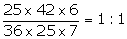5. Find the value of x, if:

(i) (2x + 3): (5x – 38) is the duplicate ratio of √5: √6.

(ii) (2x + 1): (3x + 13) is the sub-duplicate ratio of 9: 25.

(iii) (3x – 7): (4x + 3) is the sub-triplicate ratio of 8: 27.

Solution:

(i) (2x + 3): (5x – 38) is the duplicate ratio of √5: √6

And, the duplicate ratio of √5: √6 = 5: 6

So,

(2x + 3)/ (5x – 38) = 5/6

12x + 18 = 25x – 190

25x – 12x = 190 + 18

13x = 208

x = 208/13 = 16

(ii) (2x + 1): (3x + 13) is the sub-duplicate ratio of 9: 25

Then the sub-duplicate ratio of 9: 25 = 3: 5

(2x + 1)/ (3x + 13) = 3/5

10x + 5 = 9x + 39

x = 34

(iii) (3x – 7): (4x + 3) is the sub-triplicate ratio of 8: 27

And the sub-triplicate ratio of 8: 27 = 2: 3

(3x – 7)/ (4x + 3) = 2/3

9x – 8x = 6 + 21

x = 27

6. What quantity must be added to each term of the ratio x: y so that it may become equal to c: d?

Solution:

Let’s assume the required quantity which has to be added be p.

So, we havedx + pd = cy + cp

pd – cp = cy – dx

p(d – c) = cy – dx

p = cy –dx/ (d – c)

7. A woman reduces her weight in the ratio 7: 5. What does her weight become if originally it was 84 kg?

Solution:

Let’s consider the woman’s reduced weight as x.

Given, the original weight = 84 kg

So, we have

84: x = 7: 5

84/x = 7/5

84 x 5 = 7x

x = (84 x 5)/ 7

x = 60

Therefore, the reduced weight of the woman is 60 kg.

8. If 15(2x2 – y2) = 7xy, find x: y; if x and y both are positive.

Solution:

15(2x2 – y2) = 7xyLet’s take the substitution as x/y = a

2a – 1/a = 7/15

(2a2 – 1)/ a = 7/15

30a2 – 15 = 7a

30a2 – 7a – 15 = 0

30a2 – 25a + 18a – 15 = 0

5a(6a – 5) + 3(6a – 5) = 0

(6a – 5) (5a + 3) = 0

So, 6a – 5 = 0 or 5a + 3 = 0

a = 5/6 or a = -3/5

As, a cannot be taken negative (ratio)

Thus, a = 5/6

x/y = 5/6

Hence, x: y = 5: 6

9. Find the:

(i) fourth proportional to 2xy, x2 and y2.

(ii) third proportional to a2 – b2 and a + b.

(iii) mean proportional to (x – y) and (x3 – x2y).

Solution:

(i) Let the fourth proportional to 2xy, x2 and y2 be n.

2xy: x2 = y2: n

2xy × n = x2 × y2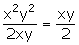n =

(ii) Let the third proportional to a2 – b2 and a + b be n.

a2 – b2, a + b and n are in continued proportion.

a2 – b2: a + b = a + b: n

n =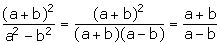(iii) Let the mean proportional to (x – y) and (x3 – x2y) be n.

(x – y), n, (x3 – x2y) are in continued proportion

(x – y): n = n: (x3 – x2y)

n2 = (x -y) (x3 – x2y)

n2 = (x -y) x2(x – y)

n2 = x2 (x – y)2

n = x(x – y)

10. Find two numbers such that the mean proportional between them is 14 and third proportional to them is 112.

Solution:

Let’s assume the required numbers be a and b.

Given, 14 is the mean proportional between a and b.

a: 14 = 14: b

ab = 196

a = 196/b …. (1)

Also, given, third proportional to a and b is 112.

a: b = b: 112

b2 = 112a …. (2)

Using (1), we have:

b2 = 112 × (196/b)

b3 = 143 x 23

b = 28

From (1),

a = 196/ 28 = 7

Therefore, the two numbers are 7 and 28.

11. If x and y be unequal and x: y is the duplicate ratio of x + z and y + z, prove that z is mean proportional between x and y.

Solution:

Given,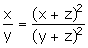x(y2 + z2 + 2yz) = y(x2 + z2 + 2xz)

xy2 + xz2 + 2yzx = x2y + z2y + 2xzy

xy2 + xz2 = x2y + z2y

xy(y – x) = z2(y – x)

xy = z2

Therefore, z is mean proportional between x and y.

12. If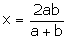, find the value of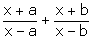.

Solution:

x = 2ab/ (a + b)

x/a = 2b/(a + b)

Applying componendo and dividendo,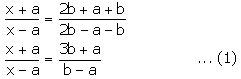Also, x = 2ab/ (a + b)

x/b = 2a/ (a + b)

Applying componendo and dividendo, we have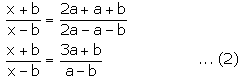Now, comparing (1) and (2) we have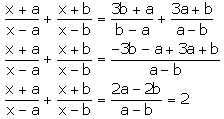13. If (4a + 9b) (4c – 9d) = (4a – 9b) (4c + 9d), prove that:

a: b = c: d.

Solution:

Given,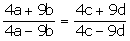Applying componendo and dividendo, we get8a/18b = 8c/18d

a/b = c/d

– Hence Proved